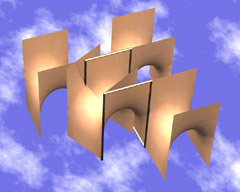# Touching Soap Films

An Introduction to Minimal Surfaces
By Hermann Karcher and Konrad Polthier

Up
Prev
Next

## Symmetry PropertiesFig 11. Applying symmetry operations to Gergonne's surface leading to a 2-periodic surface. Video (10.8MB, 2.9MB)

Using Björling's theorem, we can prove two important symmetry properties of minimal surfaces. First case: if the curve of the Strip Theorem lies in a plane and all tangent planes of the surface along the curve are perpendicular to this plane, then the strip is mirror-symmetric with respect to the plane of the strip curve. Therefore the surface continues in the same way on either side of the plane and is therefore mirror-symmetric with respect to the plane. An example of this situation is the waist-strip of the aforementioned catenoid, or can be seen in Fig 20.

Second case: an additional symmetry property is generated similarly, if a straight line segment lies in the surface. In that case the strip containing the line segment is rotated by 180° about the segment, and as a consequence the complete minimal surface itself must be symmetrical in the same way (cf the straight lines in the helicoid in Fig 10 and in Gergonne's surface in Fig 11).

A piece of a minimal surface can contain several line segments, as is shown by the Gergonne surface (Fig 8), whose boundary curve consists of a chain of orthogonal line segments. The finite minimal surface bounded by this curve can be reflected at each of these boundary segments and continued beyond them. Through further reflections at the newly created boundary segments, the surface can be extended to infinity. If one applies these same symmetry operations (reflection at each of the straight boundary segments) to the square prism of whose edges each boundary segment is composed, infinitely many specimens of the square prism-each incident on another-are generated, with no overlapping. Every prism face borders an empty space of the same shape. As a result of this agreeable property of the symmetry operations, the complete minimal surface is also free of self-intersections.